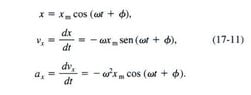# Omission of parts of equations in solving oscillation questions

• Student084
In summary: There is damping in the second problem I linked. Anyway, I understood your explanation. Thank you very...very much.

#### Student084

Homework Statement
Omission of parts of equations in solving oscillation questions.
Relevant Equations
a(t)=W² Xm cos(wt+ϕ)
x(t)= Xm e^(-bt/2m) cos(w't+ϕ)
Perhaps that's a very dumb question, but I'm having a hard time to understand why it's possible to omit parts of the equations in solving various problems involving oscillations. Here, for example, the complete equation for acceleration is not used (the part with cosine doesn't appear) and here the complete equation for displacement in damped oscillations (the part with cosine doesn't appear) is also not used.

For the first one, I do not understand where you think a cosine term has been omitted. If you write x(t) in terms of sines and cosines then differentiate twice you get ##\ddot x+\omega^2x=0##, and that is the equation used to solve the problem.

In the second, we are only concerned with a number of whole cycles. In a ratio of displacements at such times, the cosine terms are the same and cancel.

Student084 said:
Relevant Equations:: a(t)=W² Xm cos(wt+ϕ)
x(t)= Xm e^(-bt/2m) cos(w't+ϕ)
The general solution to the oscillator differential equation (without damping i.e. b=0) is given by $$x(t)=A\cos( \omega t+\phi)$$ This contains two constants ##\phi,A## which are determined by initial conditions which particularize the solution to your problem at hand. All the other forms of the solution (with sin and cos or complex numbers) are Algebraic rearrangements of the above...no new physics, just some trig and each has two undetermined constants.

haruspex said:
For the first one, I do not understand where you think a cosine term has been omitted. If you write x(t) in terms of sines and cosines then differentiate twice you get ##\ddot x+\omega^2x=0##, and that is the equation used to solve the problem.

In the second, we are only concerned with a number of whole cycles. In a ratio of displacements at such times, the cosine terms are the same and cancel.
I understood your explanation for the second question, but my physics textbook shows the equation for acceleration with a cosine term (image attached), so I still don't understand why it doesn't appear in the equation used to solve the first question.

#### Attachments

•864864.JPG
8.2 KB · Views: 49
Last edited:
Student084 said:
I understood your explanation for the second question, but my physics textbook shows the equation for acceleration with a cosine term (image attached), so I still don't understand why it doesn't appear in the equation used to solve the first question.
There are two ways you can write the same equation
##a=-\omega^2~x##
##a=-\omega^2~x_m\cos(\omega t+\phi)##

Is that what troubles you?

kuruman said:
There are two ways you can write the same equation
##a=-\omega^2~x##
##a=-\omega^2~x_m\cos(\omega t+\phi)##

Is that what troubles you?
Unless we are talking about a moment when the displacement is maximum and therefore the cosine term is equal to 1, I don't understand how these two equations can be the same

We are in a semantic impasse. When I say the "equation of motion" I mean $$x(t)=A\cos( \omega t+\phi)$$ (with the two constants specified and ##\omega## presumed known). It contains all information about the motion of the particle.
One could report in infinite number of derivatives (v(t), a(t),...) without increasing the information content. Why stop at three? Your book should be clearer about this. Differential calculus immediately supplies the rest.

Student084 said:
Homework Statement:: Omission of parts of equations in solving oscillation questions.
Relevant Equations:: a(t)=W² Xm cos(wt+ϕ)
x(t)= Xm e^(-bt/2m) cos(w't+ϕ)

Perhaps that's a very dumb question, but I'm having a hard time to understand why it's possible to omit parts of the equations in solving various problems involving oscillations. Here, for example, the complete equation for acceleration is not used (the part with cosine doesn't appear) and here the complete equation for displacement in damped oscillations (the part with cosine doesn't appear) is also not used.
Hello @Student084 .There is no damping in this problem, so skip the exponential factor in your Relevant Equation. Also, That's an omega (ω), but if you use the letter, w, instead it should be lower case every where.

So you have: x(t) = xmax cos(ωt + ϕ)

and: a(t) = −ω2 xmax cos(ωt + ϕ)

So at any particular time, t, you have that

a(t) = −ω2 x(t) .

It merely a substitution.

SammyS said:
Hello @Student084 .There is no damping in this problem, so skip the exponential factor in your Relevant Equation. Also, That's an omega (ω), but if you use the letter, w, instead it should be lower case every where.

So you have: x(t) = xmax cos(ωt + ϕ)

and: a(t) = −ω2 xmax cos(ωt + ϕ)

So at any particular time, t, you have that

a(t) = −ω2 x(t) .

It merely a substitution.
There is damping in the second problem I linked. Anyway, I understood your explanation. Thank you very much.